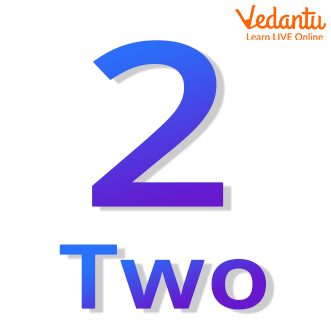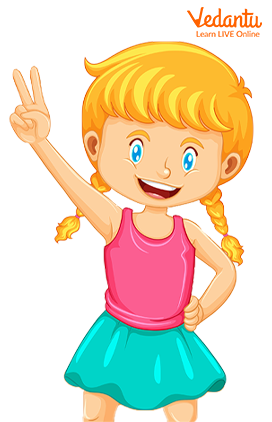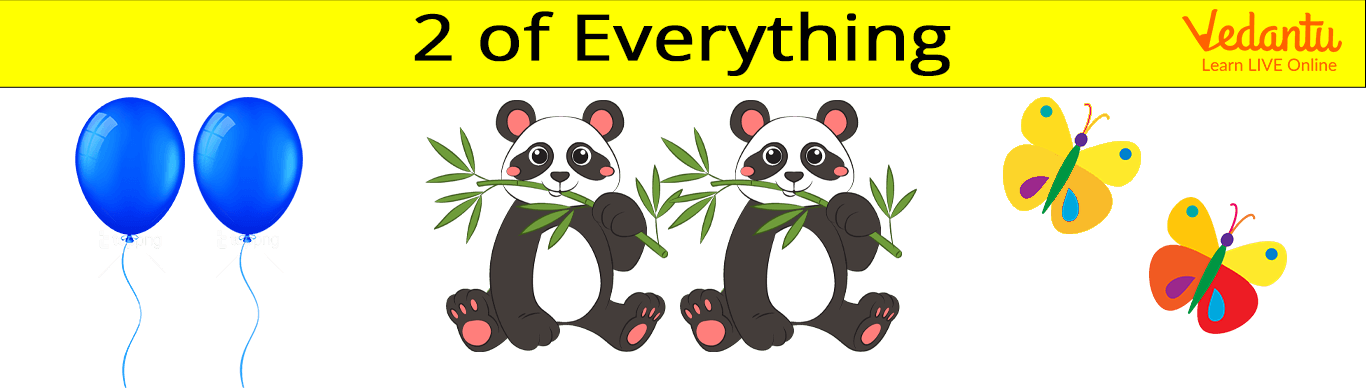Courses
Courses for Kids
Free study material
Free LIVE classes
More

# How to Write and Learn Number 2?## An Introduction to the Number 2 for Kids

We all look at various things around us, some of them are easily countable while others may not be so easy to quantify. Things like the number of butterflies, the wheels of a car, and the number of family members are easily countable while the amount of water in the sea or the number of stars is not countable. As kids are curious about the environment around them and like to understand the natural process, introducing them to the concept of quantification can be fun and help them excel academically. But to familiarise kids with the concept of counting, they must first know how to quantify things.

Numbers are used to quantify various objects. The number is a mathematical term that is used for the quantification of the object; it can be used to measure, label, and count various things. The numbers are also used to solve arithmetic problems. In this article, we answer the question: what is 2? We also provide some tips on how kids can write and memorise the number two.

## What is 2?

Since we have understood what a number is, let us answer the question: what is 2? Well, 2 is a number that represents the quantity and value of the two. The number two is a whole number that is preceded by 1 and is succeeded by 3 and then 4. Given below is an image of how the number is represented in words and the number form in Mathematics.Image: Illustration of the number two

## How to Count the Number?

As we learnt what the number 2 is, let us now understand how to count the number. The counting starts from 1. The number 1 is then followed by 2, then 3, then 4, and then 5, 6, 7, 8, 9, and finally 10. Let us look into some of the pictorial examples of what is 2, what does it represent.Image: A girl holding up fingers

The image given above shows the girl holding 2 fingers. The figures represent the number 2. Let us look into some other examples of what is 2.Image: Two balloons, pandas, and butterflies

The image shows 2 balloons, 2 pandas, and 2 butterflies.

## How to Memorise What is 2?

Learning the numbers and using them to count things in the practical world is a very interesting activity. It can be fun for children while also allowing them to develop their basics of Mathematics. A fun activity that can help kids to learn what is 2 is to sing along with a song that helps kids to memorise the number. An example of such a song is mentioned below.

2 pretty birds fly in the sky,

2 yellow balloons fly with the high,

They fly Up! Up! And up so high!

Little kids wave them goodbye!

## How to Write 2 in Words?

Learning numbers is fun!! Let us learn how to write 2 in words.

2 is spelt as TWO.

Image: A girl shows how to write 2 in words

It is important to learn how to write 2 in words, as it can be used in a sentence. For example, if one has 2 candies, he or she can write they have two candies.

Since we have learnt what is 2 and how to write in words, we can now conclude the article. Learning new things and applying them to the things around is always fun. A small tip for parents is to engage their kids in the learning process in a playful manner. Learning things with a natural flow can help kids to better understand the concepts and maintain their curiosity about the concepts.

Last updated date: 26th Sep 2023
Total views: 137.7k
Views today: 1.37k

## FAQs on How to Write and Learn Number 2?

1. How to write two in the place value chart.

A place value chart in Maths is a system that represents the value of the number. In place value identification, the number is written after identifying the place for each digit in the given number. In the case of 2, we can observe that the digit in a number is equal to the unit place.

2. Give an example of where the value of a thing is two.

There are various such examples where the quantification can result in 2. For example, humans have 2 hands, 2 ears, and 2 legs. There are two wheels on the bicycle. Dogs have 2 eyes and birds have 2 wings.